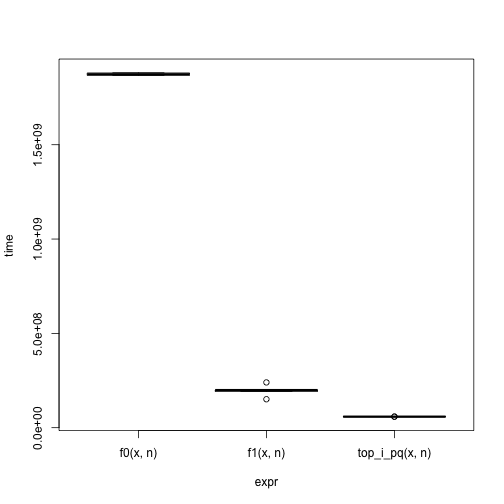# Extended example: Rcpp

Indices of the largest n elements of a large vector (from StackOverflow).

Ideas??

# Implementations

## R solutions

Sort in decreasing order, finding the nth largest, then scan for values >= the largest value.

f0 <- function(x, n)
which(x >= sort(x, decreasing=TRUE)[n], arr.ind=TRUE)


Partially sort, accomodating the limitation that partial sorting is only supported in increasing order

f1 <- function(x, n)
which(x >= -sort(-x, partial=n)[n], arr.ind=TRUE)


## An Rcpp / Standard Template Library solution

Start by including the appropriate headers and using namespaces for convenience

#include <Rcpp.h>
#include <queue>

using namespace Rcpp;
using namespace std;


Arrange to expose the C++ function to R

// [[Rcpp::export]]
IntegerVector top_i_pq(NumericVector v, int n)


and define some variables, most importantly a priority_queue to hold as a pair the numeric value and index. The queue is ordered so the smallest values are at the 'top', with small relying on the standard pair<> comparator.

typedef pair<double, int> Elt;
priority_queue< Elt, vector<Elt>, greater<Elt> > pq;
vector<int> result;


Walk through the input data, adding it to the queue if either (a) there are fewere than n values in the queue or (b) the current value is larger than the smallest value in the queue. In the latter case, pop off the smallest value, and insert it's replacement. In this way the priority queue always contains the n largest elements.

for (int i = 0; i != v.size(); ++i) {
if (pq.size() < n)
pq.push(Elt(v[i], i));
else {
Elt elt = Elt(v[i], i);
if (pq.top() < elt) {
pq.pop();
pq.push(elt);
}
}
}


Finally pop the indexes from the priority queue into the return vector, remembering to translate to 1-based R coordinates.

result.reserve(pq.size());
while (!pq.empty()) {
result.push_back(pq.top().second + 1);
pq.pop();
}


and return the result to R

return wrap(result);


The full code is available at

system.file(package="UnderstandingRBioc", "extdata", "top_i_pq.cpp")


This has nice memory use (the priority queue and return vector are both small relative to the original data) and should be fast.

Problems with this code include:

1. The default comparator greater<Elt> works so that, in the case of a tie spanning the value of the _n_th element, the last, rather than first, duplicate is retained.

2. NA values (and non-finite values?) may not be handled correctly; I'm not sure whether this is true or not (they are handled correctly, though perhaps more by accident than by design).

3. The function only works for NumericVector input, but the logic is appropriate for any R data type for which an appropriate ordering relationship is defined.

These issues have been taken up, with a faster and more general algorithm, in an Rcpp Gallery post.

## Output and performance

library(microbenchmark)
library(Rcpp)

fl <- system.file(package="UnderstandingRBioc", "extdata",
"top_i_pq.cpp")
sourceCpp(fl)

n <- 10000
x <- rnorm(1e7)

res0 <- f0(x, n); res1 <- f1(x, n); res2 <- top_i_pq(x, n)
identical(res0, res1)

##  TRUE

identical(res0, res2)

##  FALSE

identical(res0, sort(res2))

##  TRUE


times <- microbenchmark(f0(x, n), f1(x, n), top_i_pq(x, n), times=5)
print(times)

## Unit: milliseconds
##            expr     min      lq  median      uq     max neval
##        f0(x, n) 1867.78 1867.78 1873.44 1880.63 1881.28     5
##        f1(x, n)  150.95  191.95  199.36  199.59  240.00     5
##  top_i_pq(x, n)   57.72   58.35   58.38   58.57   59.22     5

plot(times)## Packaging

Shared libraries are often most useful when incorporated into R packages. Packages make it easy to distribute shared libraries for re-use by colleagues, to document functionality in a way that is accessible to non-C++ programmers, and to test the code across platforms. Create the skeleton of an Rcpp package with

Rcpp.package.skeleton("CppTest")


This produces the basic structure of an Rcpp package in a directory CppTest; the details need to be completed as outlined in the file CppTest/Read-and-delete-me. The DESCRIPTION file includes the line

Depends: Rcpp (>= 0.10.4)


so that the Rcpp package (especially headers) are available when the package is built and installed. The NAMESPACE file indicates that a shared library is present and will be used:

useDynLib(TestCpp)


The src/Makevars and src/Makevars.win (for Windows) files. The Makevars files configure the compiler to find Rcpp headers:

PKG_LIBS = $(R_HOME)/bin/Rscript -e "Rcpp:::LdFlags()"  The src/rcpp_hello_world.h contains the line RcppExport SEXP rcpp_hello_world();  to indicate that the function rcpp_hello_world will be visible from within R. If linking to additional libraries is required, add lines like PKG_CPPFLAGS=-I/opt/boost_1_54_0/include -I../inst/include PKG_LIBS = -L/opt/boost_1_54_0/lib -lboost_regex \ $(R_HOME)/bin/Rscript -e "Rcpp:::LdFlags()"


to Makevars.